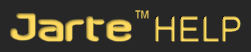Equation EditorContents   |    Search   |    Support   |    Jarte Home
 Advanced setting Equation File Ext is used to determine the equation editor program Jarte associates with its Insert Equation command. If the setting is left blank Jarte will use the MathType equation editor for inserting equations if that program is installed. The value of this setting is the file name extension of the equation files produced by the desired equation editor. For example: Equation File Ext=mmf "mmf" is the file name extension of equation files created using the MathMagic equation editor. The above setting will cause the Insert Equation command to start the MathMagic program to create a new equation instead of looking for the MathType equation editor (assuming MathMagic is installed). The file name extension used by an equation editor program can usually be determined by selecting Save As in the program and looking at the Save As Type field. The specified file name extension must be an extension specific to the equation editor program (i.e., the extension can not be a generally used extension such as EPS, WMF, JPG, or GIF). The default is to leave the setting blank: Equation File Ext= How to Change an Advanced Setting See Advanced Settings for details on how to find and change the value of a Jarte advanced setting.Carolina Road Software